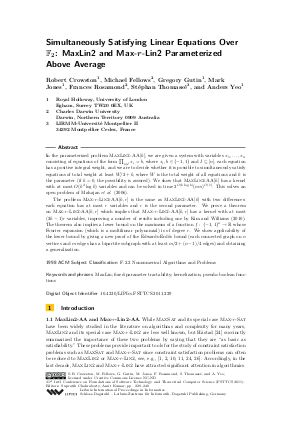Document# Simultaneously Satisfying Linear Equations Over F_2: MaxLin2 and Max-r-Lin2 Parameterized Above Average

### Authors Robert Crowston, Michael Fellows, Gregory Gutin, Mark Jones, Frances Rosamond, Stéphan Thomassé, Anders Yeo## File

LIPIcs.FSTTCS.2011.229.pdf
• Filesize: 0.48 MB
• 12 pages

## Cite As

Robert Crowston, Michael Fellows, Gregory Gutin, Mark Jones, Frances Rosamond, Stéphan Thomassé, and Anders Yeo. Simultaneously Satisfying Linear Equations Over F_2: MaxLin2 and Max-r-Lin2 Parameterized Above Average. In IARCS Annual Conference on Foundations of Software Technology and Theoretical Computer Science (FSTTCS 2011). Leibniz International Proceedings in Informatics (LIPIcs), Volume 13, pp. 229-240, Schloss Dagstuhl - Leibniz-Zentrum für Informatik (2011)
https://doi.org/10.4230/LIPIcs.FSTTCS.2011.229

## Abstract

In the parameterized problem MaxLin2-AA[\$k\$], we are given a system with variables x_1,...,x_n consisting of equations of the form Product_{i in I}x_i = b, where x_i,b in {-1, 1} and I is a nonempty subset of {1,...,n}, each equation has a positive integral weight, and we are to decide whether it is possible to simultaneously satisfy equations of total weight at least W/2+k, where W is the total weight of all equations and k is the parameter (if k=0, the possibility is assured). We show that MaxLin2-AA[k] has a kernel with at most O(k^2 log k) variables and can be solved in time 2^{O(k log k)}(nm)^{O(1)}. This solves an open problem of Mahajan et al. (2006). The problem Max-r-Lin2-AA[k,r] is the same as MaxLin2-AA[k] with two differences: each equation has at most r variables and r is the second parameter. We prove a theorem on Max-\$r\$-Lin2-AA[k,r] which implies that Max-r-Lin2-AA[k,r] has a kernel with at most (2k-1)r variables, improving a number of results including one by Kim and Williams (2010). The theorem also implies a lower bound on the maximum of a function f that maps {-1,1}^n to the set of reals and whose Fourier expansion (which is a multilinear polynomial) is of degree r. We show applicability of the lower bound by giving a new proof of the Edwards-Erdös bound (each connected graph on n vertices and m edges has a bipartite subgraph with at least m/2 +(n-1)/4 edges) and obtaining a generalization.
##### Keywords
• MaxLin
• fixed-parameter tractability
• kernelization
• pseudo-boolean functions

## Metrics

• Access Statistics
• Total Accesses (updated on a weekly basis)
0